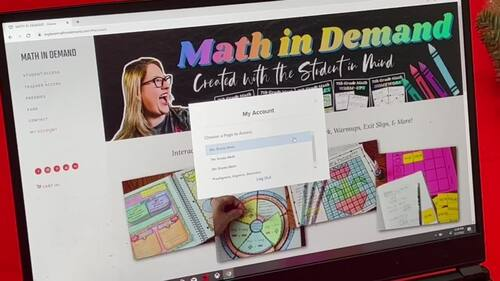# 6th Grade Math Curriculum Bundle (Interactive Notebook, Homework, Activities)

Rated 4.93 out of 5, based on 52 reviews
52 Ratings;
6th, Homeschool
Subjects
Resource Type
Standards
Formats Included
• Zip
Pages
500+
\$249.99
List Price:
\$388.40
You Save:
\$138.41
Bundle
\$249.99
List Price:
\$388.40
You Save:
\$138.41
Bundle
Report this resource to TPT

#### What educators are saying

This was very helpful in my planning. The students found many of the activities very enjoyable and engaging.

#### Products in this Bundle (16)

showing 1-5 of 16 products

### Description

⭐ This bundle includes ALL of my 6th Grade Math material in my store and ALL future 6th Grade Math Products ⭐

This includes all of my popular 6th grade math interactive notebooks, practice worksheets, foldables, activities, warm-ups, homework, early finishers, exit slips, assessments, and SO MUCH MORE!!! You can use this bundle as a stand alone curriculum for the entire school year or you can use it to supplement your current curriculum.

This bundle includes ALL of the following:

Don't miss out on this amazing deal!!! Individually this bundle costs well over \$600!

Unit 1 - Number Sense

✅ Subtracting Decimals

✅ Multiplying Decimals

✅ Dividing Decimals

✅ Dividing Fractions with Fraction Bars

✅ Dividing Fractions

✅ Word Problems involving Dividing Fractions

✅ Exponents

✅ Order of Operations

✅ Intro to Negative Numbers & Comparing Negative Numbers

✅ Positive and Negative Numbers

✅ Number Opposites

✅ Absolute Value

✅ Coordinate Plane

✅ Distance between Points with a Same Coordinate

✅ Least Common Multiple

✅ Greatest Common Factor

Unit 2 - Ratios, Rates, & Percentages

✅ Intro to Ratios & Word Problems

✅ Intro to Rates & Word Problems

✅ Intro to Percents

✅ Percent-Decimal Conversions

✅ Percent of a Number – Finding an Amount

✅ Finding a Percent

✅ Finding the Base

Unit 3 - Expressions & Equations

✅ Intro to Variables

✅ Evaluating Expressions

✅ Writing Algebraic Expressions

✅ Solving One-Step Equations

✅ Intro to Inequalities

✅ Solving One-Step Inequalities

✅ Dependent vs Independent Variables

✅ Combining Like Terms

✅ The Distributive Property

✅ Writing Equivalent Expressions

Unit 4 - Geometry

✅ Area of Parallelograms

✅ Area of Triangles

✅ Area of Trapezoids

✅ Area of Composite Figures

✅ Identifying Parts of 3-Dimensional Objects

✅ Volume of Rectangular Prisms (Fractional Lengths)

✅ Surface Area using Nets

✅ Polygons in the Coordinate Plane

Unit 5 - Statistics & Probability

✅ Dot Plots & Frequency Tables

✅ Histograms

✅ Mean, Median, Mode, and Range

✅ Box Plots (Includes IQR)

Related Products

This resource includes a limited use license from Math in Demand. You may only use the resource for personal classroom use. This purchase does not allow you to transfer my resource to another teacher, school, or etc. You must purchase an additional license at a discounted cost.

Questions? Please email me at mathindemand@hotmail.com or click the Q&A tab.

Total Pages
500+
Included
Teaching Duration
1 Year
Report this resource to TPT
Reported resources will be reviewed by our team. Report this resource to let us know if this resource violates TPT’s content guidelines.

### Standards

to see state-specific standards (only available in the US).
Find the area of right triangles, other triangles, special quadrilaterals, and polygons by composing into rectangles or decomposing into triangles and other shapes; apply these techniques in the context of solving real-world and mathematical problems.
Find the volume of a right rectangular prism with fractional edge lengths by packing it with unit cubes of the appropriate unit fraction edge lengths, and show that the volume is the same as would be found by multiplying the edge lengths of the prism. Apply the formulas 𝘝 = 𝘭 𝘸 𝘩 and 𝘝 = 𝘣 𝘩 to find volumes of right rectangular prisms with fractional edge lengths in the context of solving real-world and mathematical problems.
Draw polygons in the coordinate plane given coordinates for the vertices; use coordinates to find the length of a side joining points with the same first coordinate or the same second coordinate. Apply these techniques in the context of solving real-world and mathematical problems.
Represent three-dimensional figures using nets made up of rectangles and triangles, and use the nets to find the surface area of these figures. Apply these techniques in the context of solving real-world and mathematical problems.
Recognize a statistical question as one that anticipates variability in the data related to the question and accounts for it in the answers. For example, “How old am I?” is not a statistical question, but “How old are the students in my school?” is a statistical question because one anticipates variability in students’ ages.